Courses

# Theory & Procedure, Refractive Index of Liquid Class 12 Notes | EduRev

## JEE : Theory & Procedure, Refractive Index of Liquid Class 12 Notes | EduRev

The document Theory & Procedure, Refractive Index of Liquid Class 12 Notes | EduRev is a part of the JEE Course Physics Class 12.
All you need of JEE at this link: JEE

Our Objective:

To determine the refractive index of a liquid using convex lens by parallax method.

The Theory: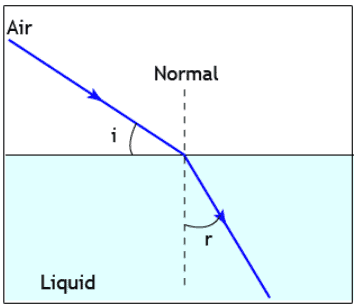In optics, the refractive index or index of refraction n of a material is a dimensionless number that describes how light propagates through that medium. It is defined as:

n = c/v

where,  c is the speed of light in vacuum and v is the phase velocity of light in the medium. For example, the refractive index of water is 1.333, meaning that light travels 1.333 times faster in a vacuum than it does in water.

The refractive index determines how much light is bent, or refracted, when entering a material. When light moves from one medium to another, it changes its direction, i.e., it is refracted.

If i is the angle of incidence of a ray in vacuum and r is the angle of refraction, the refractive index n is defined as the ratio of the sine of the angle of incidence to the sine of the angle of refraction.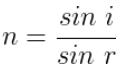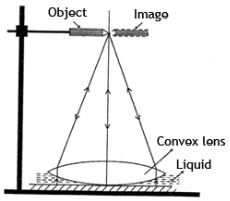When a convex lens is placed over some drops of the given liquid on a plane mirror, a plano-concave liquid lens is formed between the lens and the mirror.

If f1 is the focal length of the convex lens and F is the focal length of the combination of convex lens and plano-concave liquid lens, the focal length of the liquid lens f2 is given by,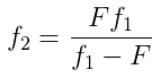If R is the radius curvature of the face of the convex lens which is in contact with the liquid, then the refractive index of the liquid is,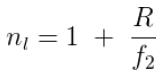Learning outcomes:

• Students understand the terms refractive index and plano–concave liquid lens.
• Students will be better able to do the experiment in a real laboratory by understanding the procedure.

Materials required:

• The given convex lens
• The given liquid- water
• Plane mirror
• Retort stand
• Pointer
• Mercury
• Meter scale
• China dish

Real Lab Procedure:

To find the focal length of the convex lens:

• The plane mirror is placed horizontally on the base of the retort stand with its reflecting surface upwards.
• The given convex lens is placed over the plane mirror.
• The pointer is arranged horizontally on the clamp of the retort stand, vertically above the lens.
• Looking from above, the height of the pointer is adjusted such that the inverted image of the pointer is obtained.
• The height is further adjusted so that the image coincides with tip of the pointer without parallax. The image and object will be of the same size.
• The distance of the pointer from the top (y1) and bottom (y2) of the lens are measured. The average of these values gives the focal length of the f1 of the convex lens.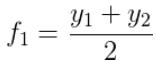To determine the focal length of the combination of convex lens and liquid lens:

• Remove the lens and place a few drops of the given liquid on the plane mirror.
• The lens is then placed over the liquid with its marked face in contact with the liquid.
• A plano-concave liquid lens is thus formed between the convex lens and the plane mirror.
• The pointer adjustment for coincidence is done and the distance from the top (y1) and bottom (y2) of the lens are measured.
• The average of the two values gives the focal length of the combination, F.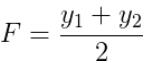• Then calculate the focal length of the liquid lens using the formula,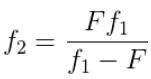To determine the radii of curvature of the lens:

• The convex lens is floated in mercury and taken in a china dish with its marked face in contact with mercury.
• The pointer adjustment for coincidence is done and the distance from the top, d1  of the lens is measured.
• The distance d of the pointer from the centre of the lens is calculated using the formula,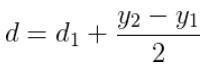• Where y2 - y1 is the thickness of the lens.
• Then calculate the radius of curvature of the lens using the formula,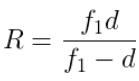• So the refractive index of the given liquid can be calculated used using the formula,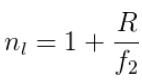• Simulator Procedure (as performed through the Online Labs)
• Select the convex lens from the drop down list.
• Select the method from the drop down list.

Without Liquid

• Select the distance of the pointer from the bottom of the lens using the slider (Object pointer).
• You can see that the size of the image varies with distance.
• Adjust the pointer so that the image coincides with the tip of the object without parallax. At this stage, the image and object will be of the same size.
• You can see the zoomed view of the object (left) and image (right) on the right side.
• You can view the object and image from different angles of view (left, centre and right) using the slider.
• Measure the height of the pointer from the bottom of the lens. It is taken as y2 cm.
• The thickness of the lens is t cm.
• You can calculate the distance of the pointer from the top of the lens (y1) using the equation, y1 = (y2 -t) cm.
• You can calculate the focal length (f1) of the convex lens using the equation,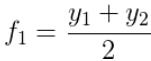• You can verify your result by clicking on the “Show result’ button.

With Liquid

• Select the liquid from the drop down list.
• Select the distance of the pointer from the bottom of the lens using the slider (Object pointer).
• You can see that the size of the image varies with the distance.
• Adjust the pointer so that the image coincides with the tip of the object without parallax. At this stage, the image and object will be of the same size.
• You can see the zoomed view of the object (left) and image (right) on the right side.
• You can view the object and image from different angles of view (left, centre and right) using the slider.
• Measure the height of the pointer from the bottom of the lens. It is taken as y2 cm.
• The thickness of the lens is fixed as t cm.
• You can calculate the distance of the pointer from the top of the lens (y1) using the equation, y= (y2 -t) cm.
• You can calculate the focal length (F) of the convex lens using the equation,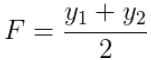• You can calculate the focal length of the liquid lens (f2) using the equation,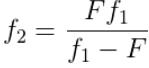• The radius of curvature of the lens is R cm.
• You can calculate the refractive index of the liquid using the equation,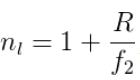• You can verify your results by clicking on the ‘Show result’ button.
Offer running on EduRev: Apply code STAYHOME200 to get INR 200 off on our premium plan EduRev Infinity!

## Physics Class 12

204 videos|288 docs|125 tests

,

,

,

,

,

,

,

,

,

,

,

,

,

,

,

,

,

,

,

,

,

,

,

,

;